An experimental system for reconstructing a scene from the image sequences produced by a moving camera

Jiri Walder,

jiri.walder.fei@vsb.cz

Technical University of Ostrava

Ostrava / Czech Republic

# Abstract

In this paper, a method and an experimental computer program for reconstructing a three-dimensional static scene from the sequence of images produced by a moving camera are presented. A method for determining the camera trajectory and for reconstructing the coordinates of feature points from multiple images is proposed. The efficiency and the robustness of this method are verified experimentally. The feature points and the correspondence relation are detected automatically by a heuristic algorithm.

Keywords: scene reconstruction, camera calibration, image processing, computer vision, point tracking.

# 1. Introduction

The paper focuses on the following problem: Consider a three-dimensional static world that will be referred to as a scene. A camera moving in this world produces a sequence of images called frames. The frames are two-dimensional pixel arrays. The two-dimensional coordinates of points can be measured in the frames. The sequence of the frames is used to create a model of the scene. The three-dimensional coordinates (in a global reference system) of the feature points that are visible in the frames are computed.

The solution to the problem of reconstructing a scene from a pair of stereo images is known for a long time . The reconstruction from three and multiple images was later studied from various viewpoints . The difficult problem involved in reconstruction is to detect the correspondence (we say that in different frames, the images of a unique point of a scene correspond to one another). Finding the correspondence by a computer algorithm is hard: (1) In order to achieve a good accuracy of reconstruction, the images should be taken from camera positions that are distant enough. (2) As the distance between the camera positions increases, the images become more and more different and the correspondence is more and more difficult to find automatically. If a whole sequence of frames produced by a moving camera is available, the problem becomes easier. Since the change between two consecutive frames is usually small, the correspondence relation for the consecutive frames can be found easily. Also, the effects of occluding can be detected reliably. The correspondence relation for non-consecutive frames can be determined as the product of the relations for the consecutive frames. We suppose that the sequence of frames is long enough. From this sequence, a certain set of significant frames can be chosen for reconstruction.

In this paper, a method and an experimental computer program for reconstructing a scene from the sequence of images produced by a moving camera are presented. A method for determining the camera trajectory (i.e., for calibrating the camera) and for reconstructing the coordinates of points of interest from multiple images is proposed. The system performing the scene reconstruction consists of the following parts: (1) Detecting the points of interest in the particular frames. (2) Tracking the points in the sequence of frames. (3) Determining the camera trajectory. (4) Reconstructing the coordinates of the points of interest. Figure 1 shows the block scheme of the system.

The paper is organised as follows: In Section 2, the model of the camera and camera motion is described. The method for calibrating the camera and the method for reconstructing the coordinates of points are presented in Sections 3.1 and 3.2, respectively. In Sections 3.3 and 3.4, the problems of detecting and tracking the points of interest are discussed.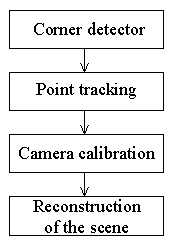Figure 1. The block scheme of the system.

# 2. The model of the camera

The camera model considered is the classical pinhole model. The basic assumption is that the relationship between the world coordinates and the pixel coordinates is linear projective. We suppose that the possible non-linear distortions of the images are corrected in advance, before the images are used for calibration and for reconstruction. As the camera moves in a three-dimensional scene, it produces a sequence of frames. We suppose that the sequence I0,I1,...,In of significant frames taken in times t0,t1,...,tn is available for calibration and for reconstruction. The sequence need not include all the frames produced by the camera. Instead, we may wait until the changes in the frames are large enough to give new information. The information in the dropped frames is then used indirectly (Section 3.3). Let (O,x,y,z) be a global coordinate system in the scene (Figure 2). For each ti, a camera coordinate system (Oi,xi,yi,zi) is considered (Oi is the centre of projection, zi is the optical axis of the camera). Let X be a point in the scene, let x=(x,y,z)T and xi=(xi,yi,zi)T represent X in (O,x,y,z) and (Oi,xi,yi,zi), respectively. We have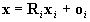, (1)
where Ri describes the rotation of (Oi,xi,yi,zi) into (O,x,y,z), and oi represents Oi in (O,x,y,z). We define Ri,j,bi,j such that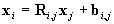. (2)
Obviously, Ri,i=I (unit matrix), and bi,i=0. It is easy to see that (we consider i<j and we define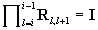)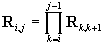, (3)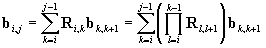. (4)
Recall that Ri,j is an orthonormal matrix and that Ri,j =Rxi,jRyi,jRzi,j. Rxi,j,Ryi,j,Rzi,j are the matrices corresponding to the rotations around the xj,yj and zj-axis, respectively. We introduce the vector ji,j = (jxi,j, jyi,j, jzi,j)T containing the rotation angles. Let fi = dist(Oi,Oi') be the focal length of the camera in ti. In each significant frame, we introduce a coordinate system (Oi',xi',yi') (Figure 2). The image coordinates are measured in a coordinate system (Zi,ui,vi). Let Xi be the image of X in the ith frame, and let ui=(ui,vi,1)T represent Xi in (Zi,ui,vi). In (Oi',xi',yi'), Xi is represented by Qiui where Qi describes the transformation of (Zi,ui,vi) into (Oi',xi',yi'). It follows that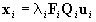, (5)
where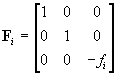,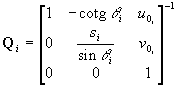. (6)
The values u0i, v0i are the coordinates (in (Zi,ui,vi)) of the point in which the optical axis pierces the projection plane. The angle qi models a possible deviation from orthogonality of the cell array of the sensor or a possible misalignment of the projection plane with respect to the optical axis. In practice, qi is usually close to p/2. The scaling factor si takes into account the possibly different sizes of the sensor cells along the u and v-axis and the distortion introduced by the frame grabber; li is a real parameter. The parameters in Fi, Qi (fi, u0i, v0i, qi, si) are the camera intrinsic parameters. The parameters describing the trajectory of the camera in space (Ri,oi or Ri,i+1,bi,i+1) are the extrinsic parameters.

# 3. The description of the system parts

## 3.1 Camera calibration

The purpose of camera calibration is to determine the intrinsic and the extrinsic parameters of the camera. Once these parameters are known, three-dimensional information can be inferred from two-dimensional images and vice versa. The calibration method proposed in this paper focuses on the problem of finding the extrinsic parameters. Thus, we suppose that Fi,Qi are known for each i. Note that a variety of methods for determining the camera intrinsic parameters were presented .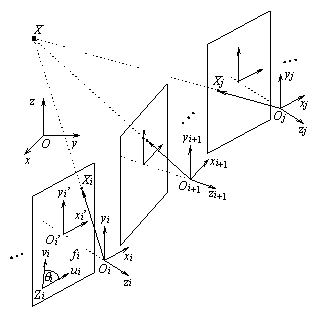Figure 1. The model of the camera and camera motion.

The information needed for calibration is provided by detecting and tracking the points of interest (Sections 3.3,3.4). In this section, we suppose that from the significant frames I0,I1,...,In, the lists of the points of interest along with their image coordinates and along with the description of the correspondence relation can be obtained. From the set of the pairs of corresponding points of interest, reliable pairs are chosen for calibration. Consider two different values ti, tj (i<j) and a point X in the scene. Let pi, pj represent the directions of lines áOiXiñ and áOjXjñ, respectively, in (Oi,xi,yi,zi). Since áOiXiñ, OjXjñ,áOiOjñ, are coplanar, the condition pi×(bi,j´pj)=0 must be satisfied. The conditions yields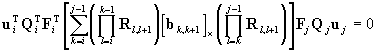. (7)
Let s = (bT0,1, jT0,1, bT1,2, jT1,2, ... , bTn-1,n, jTn-1,n)T be the vector of the values that are to be found during the calibration. Let q be the number of calibration points, let X(k) be the kth such point. We use u(k) to denote the vector of coordinates of the images of X(k) in all the frames in which X(k) is used for calibration, and, finally, we introduce the vector u = (u(1)T,  ,u(q)T )T of the image coordinates of all the calibration points. The coplanarity equation Eq.(7) can be rewritten as follows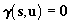. (8)
Usually, much more points than is the minimum required for calibration are detected in the frames. This can be used to reduce the influence of noise, i.e., the fact that the coordinates in the frames are measured with an error can be taken into account. Let u contain the exact coordinates. We use u0 to denote the vector of coordinates observed in the frames, and we introduce the vector Du=u-u0 of the differences and a diagonal matrix W expressing the reliability of the particular observations. The vector s then can be found by minimising the value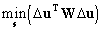. (9)
The following coplanarity equations must be satisfied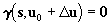. (10)
We solve the non-linear problem formulated by Eqs.(9), (10) by linearisation. Suppose that an initial estimation s0 of s is known. Let Ds be the correction of s0, i.e., s=s0 +Ds. By the Taylor expansion of Eq.(10), neglecting the higher order terms, we obtain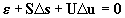, (11)
where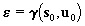,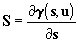,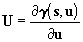. (12)
The minimisation in Eq.(9) with the condition in Eq.(11) may be solved using the method of Lagrange multipliers. Let m be the vector of Lagrange multipliers. The problem is solved by minimising the function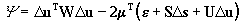. (13)
The computation yields the result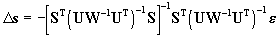. (14)
We use Eq.(14) in the iterative process. In each step of this process, we compute S,U,e and we use Eq.(14) to determine the new value of Ds. We then actualise s0 ¬s0+Ds. As a rule, only few iterations are needed (usually, no more than ten iterations). Note that in the practical implementation, we employ a heuristic deciding which points (from the set of all the points of interest detected in the frames) will be used for calibration. For the particular points, the heuristic also chooses the pairs of frames for which the coplanarity equations will be assembled. To obtain the initial value of s0, we use the classical two image approach  where the significant frames are processed in the pairs Ii,Ii+1.

Note that the multiimage calibration process described in this section can be used simultaneously (and repeatedly) with making the initial estimation of s0. Whenever the initial values of bi,i+1, ji,i+1 (for a certain i) are estimated by the two image method, the multiimage calibration process using a chosen set of significant frames can be launched to immediately improve this initial estimation.

## 3.2 Reconstruction

Providing that a point is observed in at least two significant frames, its coordinates can be reconstructed. Let X be such a point, let x be the sought vector of its coordinates in (O,x,y,z), and let ui=(ui,vi,1)T represent Xi, the image of X, in (Zi,ui,vi). Theoretically, X should lie on all the lines oi + liRiFiQiui simultaneously. If noise is present, X will not lie on the lines exactly. We measure the distances between X and the lines and minimise the sum of their squares, i.e., we carry out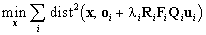. (15)
The computation yields the result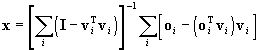, (16)
where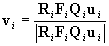. (17)

## 3.3 Detection of points of interest

The points of interest extracted from the frames both for calibration and for reconstruction are corners. The corners abound in the images of both natural and man-made scenes. They often correspond to the corners of three-dimensional objects and can be unambiguously localised in the frames. For detecting the corners, we use the Beaudet corner detector .

In 1978, P. R. Beaudet proposed the first corner detector which works directly with image intensity. The Beaudet detector locates the corners in an image at local maxima of the Gauss curvature. Firstly, Beaudets algorithm applies the operator called DET to the whole image. In this way, we obtain the image in which we search for the local maxima. The operator DET has the local maxima at positions where the corner points are situated. The maxima should be greater than a certain threshold. The operator DET is very simple and can be written as follows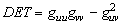, (18)
where g=g(u,v) is the input image which is represented by a two-dimensional function that describes the image intensity and guu, gvv, guv are the partial derivatives. Figure 3 shows an example of the points of interest detected in an image.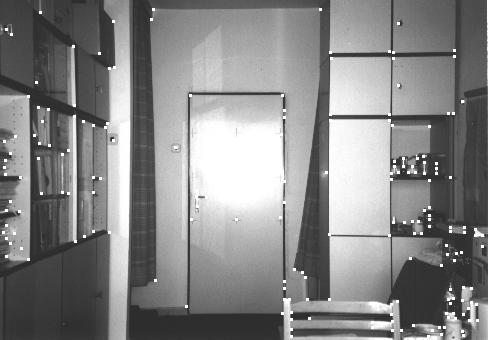Figure 3. Points of interest detected in an image.

## 3.4 Tracking the points of interest

Once a point of interest is detected in a frame, it is tracked in the subsequent frames, i.e., in the subsequent frames, we seek for the images of the same point of the scene. For tracking, we use all the frames produced by the camera, i.e., not only the significant frames. In this section, therefore, by 1,2,...,n we mean the indices in the sequence of all frames. Let ui-k,...,ui be the coordinates of the images of a point X in the frames Ii-k,...,Ii (k>0). The task of finding the coordinates ui+1 in Ii+1 of the image of X will be easier if some estimation u*i+1 of ui+1 is available. We use the Kalman filter for this purpose. Recall that in Kalman filtering, the system model, which describes the expected evolution of the vector si of state variables over time, is of the form si+1= Fi+1,isi+ Didi + Gixi. The model of measurement is zi= Hisi+hi. The values si, di, zi, xi, hi are the state vector, deterministic input, vector of measured values, process noise and measurement noise, respectively. In order to determine the system model for tracking the points in the frames we use Eqs.(2),(5). We obtain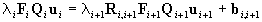. (19)
Hence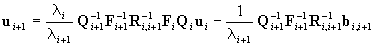. (20)
As can be easily seen, Eq.(20) is of the form that is expected by the Kalman filter. Its use, however, is not easy. In order to determine the value of ui+1, the matrices Qi+1, Fi+1, Ri,i+1 and the vector bi,i+1 are needed. These, however, are not known at the time when the value of ui+1 is to be computed. Therefore, we use an approximate model. The model is based on the assumption that the acceleration of the move of the points of interest is constant. We use two separate Kalman filters for the u,v coordinates of each point. In the following text, we restrict ourselves to the u-coordinate. The state vector is si=(ui,ui',ui'')T. The matrices of the filter are of the form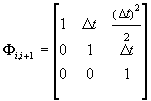, (21)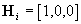,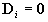, (22)
where Dt=ti+1 - ti. In our model, Gi is a diagonal matrix, constant over time, compensating for the difference between the exact and the approximate model. Note that besides predicting the coordinates of points in future frames, the Kalman filter is also used for determining the values of coordinates used for calibration and reconstruction. The filter reduces the influence of noise and gives the coordinates in sub-pixel accuracy, which improves the precision achieved in calibration and reconstruction.

The algorithm for tracking the points of interest in the sequence of frames will be presented in the following text. Consider the sequence of frames I1, I2, ..., In produced by a camera in times t = 0, 1, ..., n. The correspondence is a symmetric and transitive relation between two images of the same point. Finding all corresponding images of one point is equal to calculating the transitive closure. We implement the detection of correspondence as finding pairs of corresponding points in consecutive images It and It+1. The algorithm we propose solves this task. In time t, we find the correspondence between the points Xt and Xt+1. To understand the algorithm correctly, we have to awake that we have already found the point Xt in time t-1. In time t-1, this point was needed for finding the correspondence with the point Xt-1. The detailed description of the algorithm for finding the correspondence follows. We will describe the steps that are executed for every frame taken in time t. The algorithm consists of three sections, denoted by A, B, C.

A. Preparing the image It+1.

(1) We prepare the image It+1 for next processing. The image is read from a file produced by a camera or taken by any other form.
(2) In the image It+1, we find all the points of interest. The points of interest are detected by one of the corner detectors. Every point found in this way is inserted into a search array and is denoted by a unique index. The corresponding points have the same index.

B. Finding the filtered value and prediction

We denote by X¢t the value corrected by the Kalman filter in time t, and by X*t+1 the prediction provided by the Kalman filter in time t+1. In this section, the algorithm falls into two parts. If a new point Xt is found in the frame It, we continue by Step 3. If the point Xt is found as a point that corresponds to the point Xt-1, we continue by Step 4.
(3) If a new point is found, we initialise two Kalman filters. One for the coordinate u and the other for the coordinate v. Both the prediction X*t+1 and the filtered value X¢t are equal to the coordinates of the new point Xt.
(4) We actualise the Kalman filters for both coordinates u and v of Xt which has been found as corresponding to Xt-1. The filters provide the prediction X*t+1 and the filtered value X¢t in which the influence of noise is reduced.

C. Finding the point corresponding to Xt

(5) For point Xt, we determine a vicinity. In this vicinity, we will find the candidates for correspondence. The vicinity is a circular area whose radius is r and whose centre lies in the predicted position X*t+1. We choose the size of r to be inversely proportional to time t since as t grows, the prediction X*t+1 of the Kalman filter is more accurate. For the size of r, we can write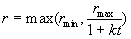. (23)
If Xt is a new point, we must choose the size of vicinity large enough to ensure reliably finding first candidates for correspondence. The starting size of the radius is rmax. Later, the size is computed by Eq. (23). The size r is never less then a minimum rmin. The parameter k determines how fast the size of vicinity decreases.
(6) All points Y that were found in the vicinity, are regarded as candidates for correspondence and the correspondence between Xt and the candidates is then checked up. In a sequence of frames, there are usually only small differences between the consequent images, hence we can say that the image functions in vicinities of corresponding points are approximately the same. Therefore, we compare the image function of a current frame in a vicinity of tracked point Xt with the image function of the consequent frame in vicinities of all candidates Y. In practice, we use a rectangle area of size w´ h. Both w and h are set to appropriate value, the recommended size is 3-9. The vicinities are compared by a function rel that gives the function values from the interval 0¸1. If the value of rel is one, the images in both vicinities are the same, if the value is zero, the images are different. Let ut, vt be the coordinates of the tracked point Xt in time t and let ut+1 and vt+1 be the coordinates of the candidate Yt+1 in time t+1. Let l denote one of the colour components. In a colour image, each pixel has three colour components r, g, b. We introduce L={r, g, b}. Suppose that the values of the colour components of pixel are chosen from the interval Jmin¸Jmax.We defined the function rel as follows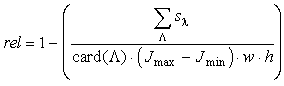, (24)
where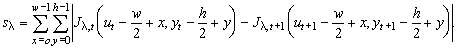(25)
The value sl is a difference of the vicinities of Xt and Yt+1, Jl,t and Jl, t+1 are the image functions in the particular frames. The function card returns the number of elements in the set L. If we know the value of rel for all candidates Y, we choose the candidate for which the value is considerably greater than for the others. If the function (24) gives the same value for two or more candidates, we choose the candidate that is closer to the predicted position X*t+1. If the value of rel is under a threshold th, we say that for the tracked point, we cannot find the corresponding counterpart. Otherwise, the point Yt+1 is declared to be a point corresponding to Xt and is given the same index as Xt. The value of th depends on the number of candidates, denoted by n. For determining th, we propose the following expression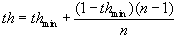, (26)
where thmin is a minimum threshold, which is chosen from the interval 0¸1.
(8) For the radius r of a scanned vicinity, we introduce an additional condition. If it happened that in Step 6, there was not found any point of interest in the scanned vicinity, the radius of vicinity is extended twice and we return back to Step 6 again. At the same time, the minimum threshold thmin is modified because we require greater reliability of function rel for candidates. Therefore, the value of thmin changes according to the expression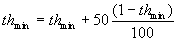. (27)
This dependence has been designed by experiments.
(9) If in the search array, any point that was not classified as corresponding remains, it is regarded as a new point. Every such point gets a unique index.
After the computation for every image It, we get an array of indexed points. We can now determine the trajectories of points for all images Ii , i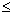t.

The above described procedure is heuristic, but our experiments have shown, that it works well. We tested this procedure on a certain number of both synthetic and real sequences. Generally, the trajectories are identified correctly. In some cases, however, certain problems may occur. It may happen, for example, that the algorithm starts to track a bad trajectory. Heuristics revealing and correcting these and similar erroneous situations are implemented. Examples of trajectories that were found in a real sequence of images are depicted in Figure 4.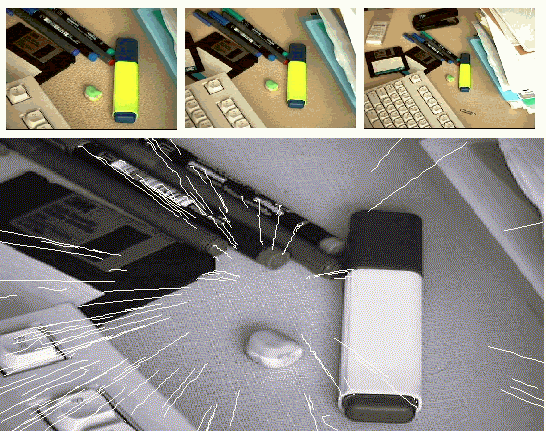Figure 4. Trajectories of points.

# 4. Conclusion

In this paper, a method and a computer program for reconstructing the three-dimensional scenes from the images produced by a moving camera were presented. A method for calibrating the camera and for reconstructing the scene from multiple images was proposed, implemented and verified experimentally. As expected, in comparison with the classical two image approach, the multiimage approach reduces the sensitivity to noise, which is the key problem in reconstruction. On the other hand, as the number of significant frames simultaneously used for calibration increases, the method becomes computationally expensive. Fortunately, only few significant frames in the time window (e.g., 3-10 frames) usually suffice to guarantee a good precision of reconstruction. Although we intended our implementation as an experimental tool and although we did not aim at constructing a real-time vision system, we are aware of the fact that the capability to work in real time is important. The current implementation, however, does not work in real time. The most computationally expensive proved to be finding and tracking the points of interest. In calibration and reconstruction, the computational load can be reduced by processing only an adequate number of significant frames in the time window.

# 5. References

  Sojka, E.: Reconstructing Three-Dimensional Objects from the Images Produced by a Moving Camera, in Proc. 8thICECGDG, Austin, Texas, USA, pp. 160-164,1998.
  Beaudet, P., R.: Rotationally Invariant Image Operators, in Proc. Fourth Int. Joint Conf. on Pattern Recognition. Tokyo, pp. 579-583, 1978.
  Daniilidis, K. and Ernst, J.: Active intrinsic calibration using vanishing points, in Pattern Recognition Letters, Vol. 17, No. 11, pp. 1179-1189, 1996.
  Ito, M. and Ishii, A.: Range and shape measurement using three-view stereo analysis, in IEEE Transactions on Pattern Analysis and Machine Intelligence, Vol. 8, No. 4, pp. 524-532., 1986.
  Longuet-Higgins, H., C.: A computer algorithm for reconstructing a scene from two projections, in Nature, Vol. 293, pp. 133-135, 1981.
  Luong, Q., T. and Faugeras, O., D.: Self-calibration of a moving camera from point correspondences and fundamental matrices, in International Journal of Computer Vision, Vol. 22, No. 3, pp. 261-289, 1997.
  Bosak, R.: Relative calibration and solving the calibration equations, Diploma thesis, Technical University of Ostrava, Czech Republic, 1998.